# Excel Array Formulas

Using standard Excel formulas on multiple cells at the same time

## What are Excel Array Formulas?

Excel array formulas allow us to use standard Excel formulas on multiple cells at the same time. Unlike standard formulas, which take individual cells as inputs, array formulas take entire arrays as inputs.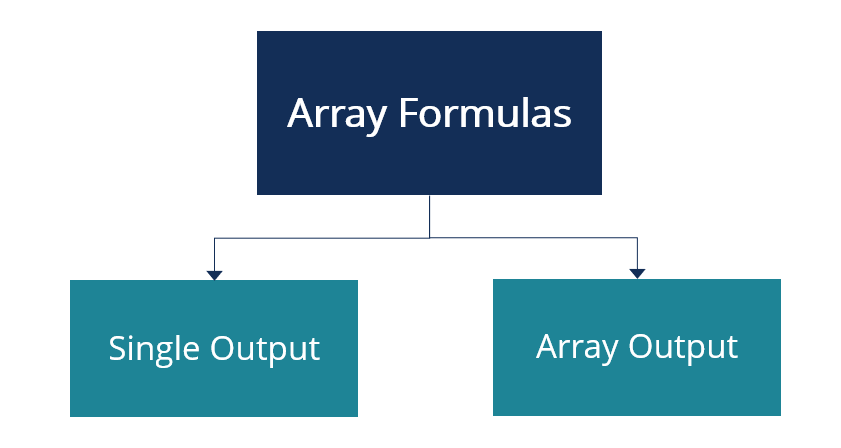### Quick Summary:

• Array formulas allow us to use standard Excel formulas on multiple cells at the same time. Unlike standard formulas, which take individual cells as inputs, array formulas take entire arrays as inputs.
• Excel uses the following format to reference arrays: First Cell:Last Cell.
• Unlike standard cell formulas that can be used by simply pressing the Enter/Return Key, array formulas in Excel are used by pressing the Control/Command, Shift, and Enter keys simultaneously.

### Types of Array Formulas

Array formulas can be broadly categorized into two groups: single output array formulas and array output array formulas.

#### 1. Single Output Array Formulas

They take a single array of data or multiple arrays of data as input and give a single cell as the output.

#### 2. Array Output Array Formulas

They take a single array of data or multiple arrays of data as input and give an array of data as the output.

### What is an Array in Microsoft Excel?

Continuous collections of cells in Microsoft Excel are known as arrays or cell arrays. Every cell in Microsoft Excel comes with a unique alphanumeric reference code, which depends solely on the position of the cell within the Excel Worksheet. Excel uses letters to measure columns and numbers to measure rows. For example, the cell in the second column and the fifth row has a cell reference of B5. Excel uses the following format to reference arrays:

First Cell:Last Cell

The cell reference of the yellow cell in the image below is B5. The cell references of the green array and red array are D1:D10 and A12:F14, respectively.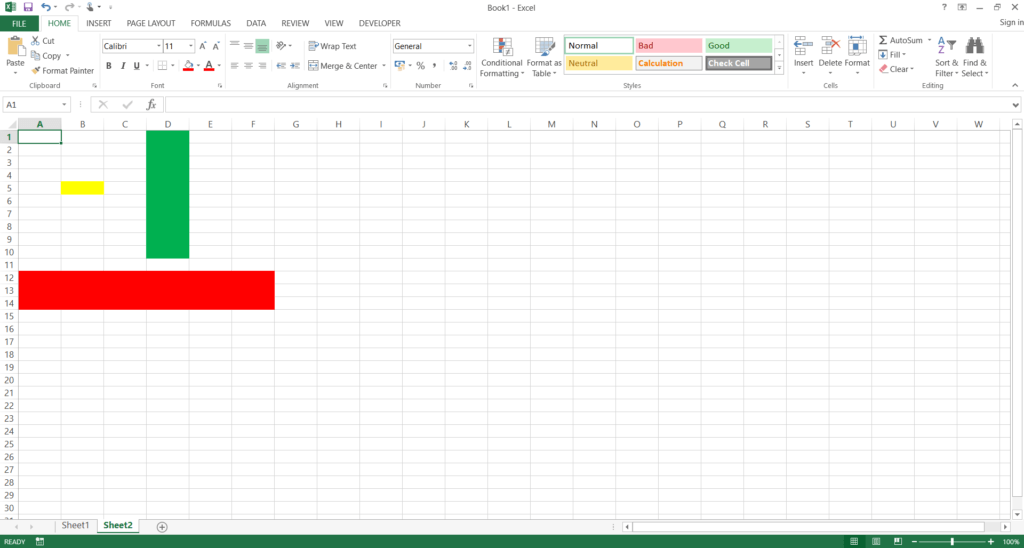### Understanding Array Formulas in Microsoft Excel

Unlike standard cell formulas that can be used by simply pressing the Enter/Return key, array formulas in Excel are used by pressing the Control/Command, Shift, and Enter keys simultaneously. If a formula is successfully recognized as an array formula, Excel automatically places the formula within curly/swirly brackets { }.

### Single Output Array Formulas – Illustrative Examples

Calculating Averages

We can find the average of an array by typing the following into Excel “=average(First Cell:Last Cell)” and pressing the Control/Command, Shift, and Enter keys simultaneously. In this case, we can find the average salary received by workers of Company ABC.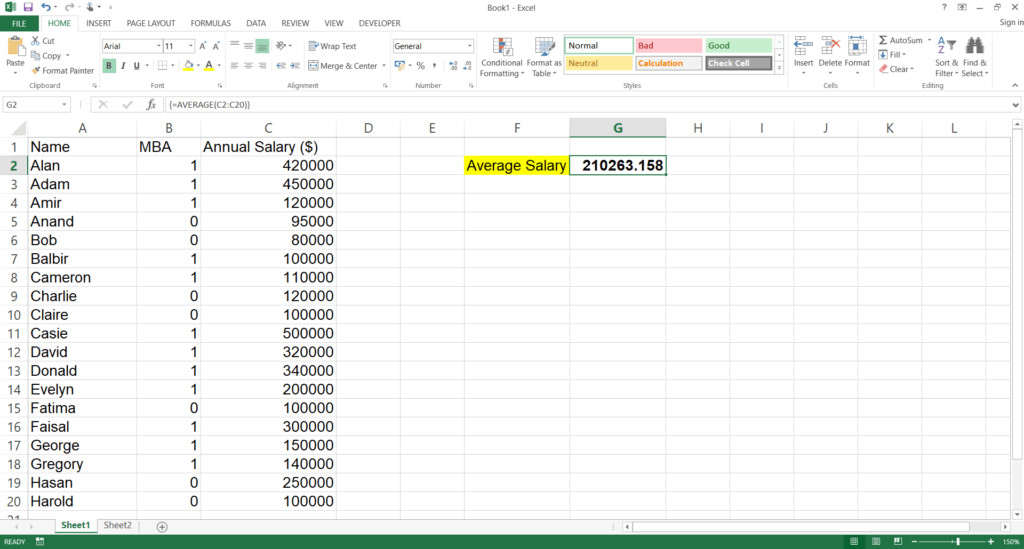Calculating Conditional Averages

We can find conditional averages using array formulas.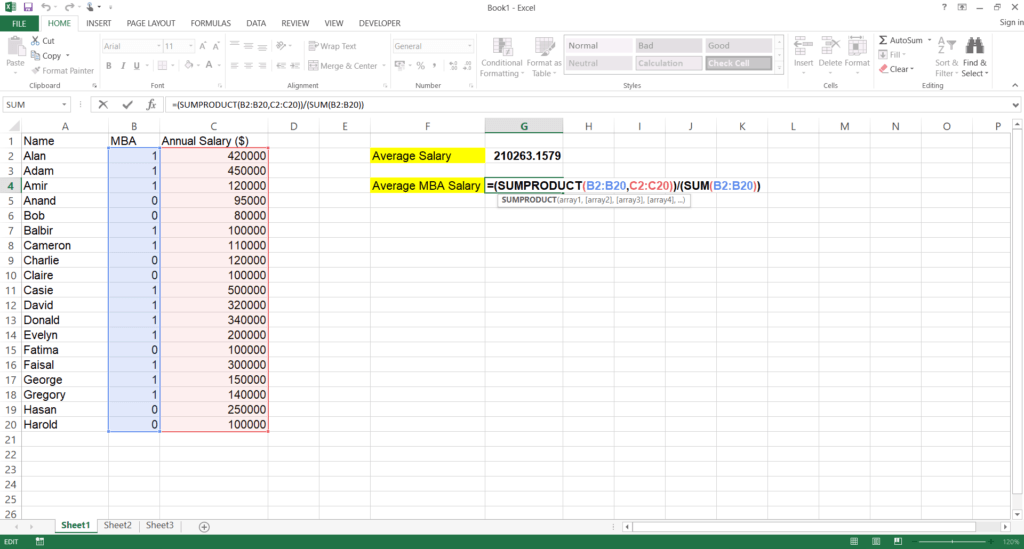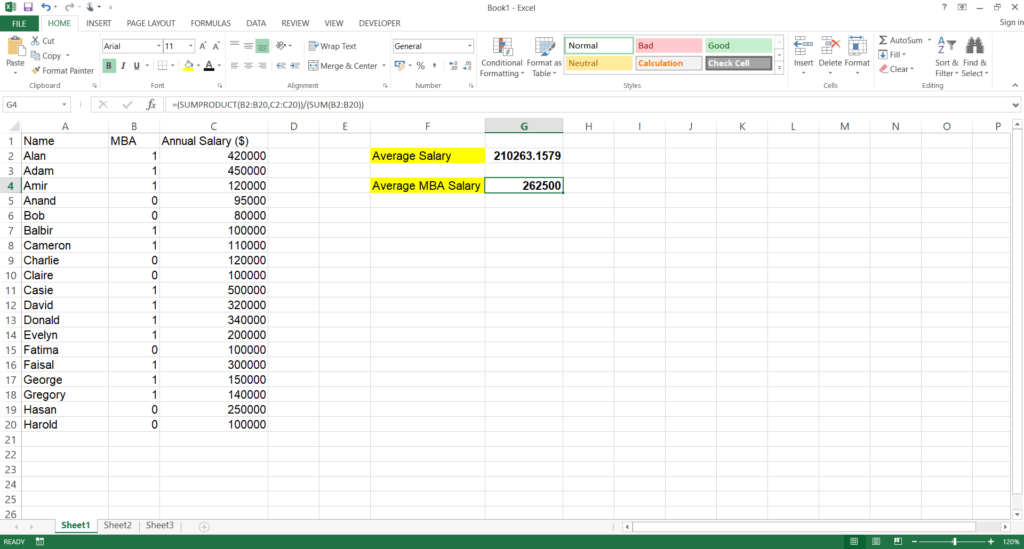### Array Output Array Formulas – Illustrative Example

Calculating Sums Across an Array

1. Suppose we are given the following data set on how much money Company ABC pays its analysts. Each analyst is paid a base salary and a discretionary bonus.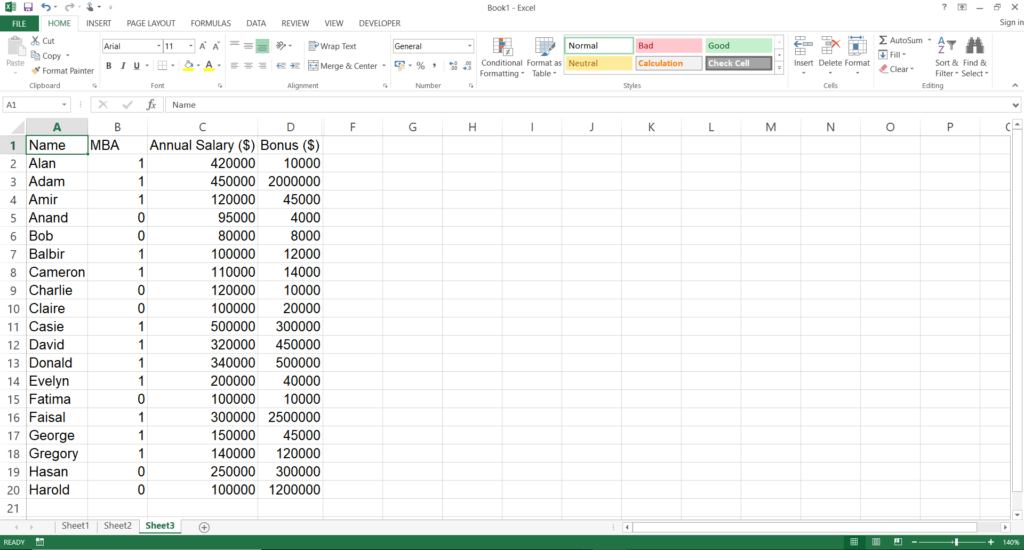2. After making a Total Pay column, we select the array where we want the output to appear in.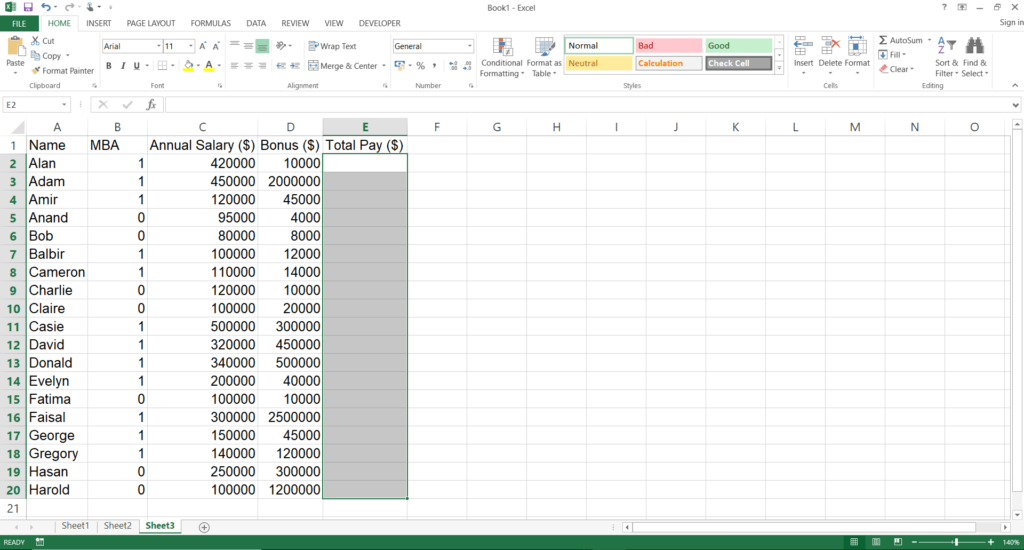3. Type the correct formula while the output array is selected.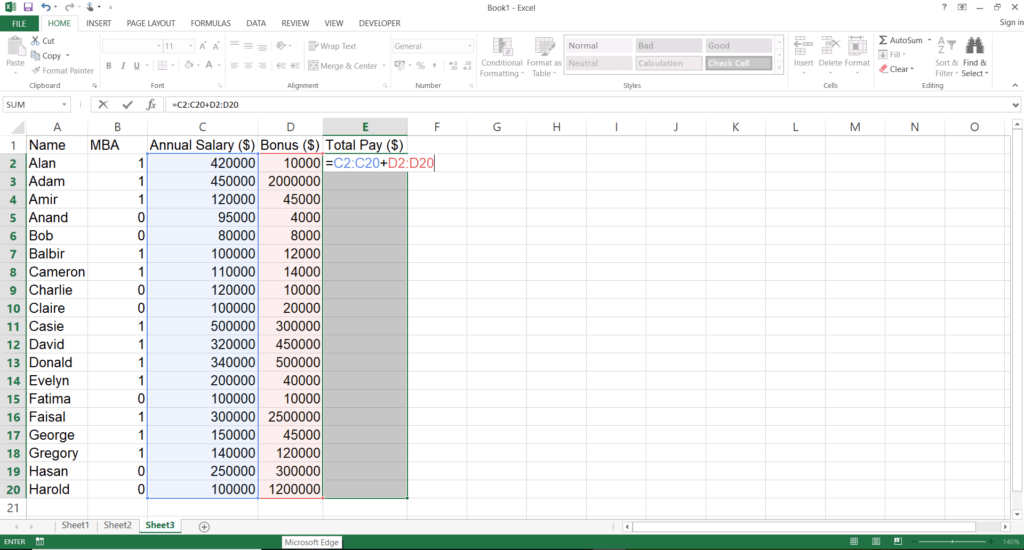4. If we only press Enter, only the first cell in the output array gets populated.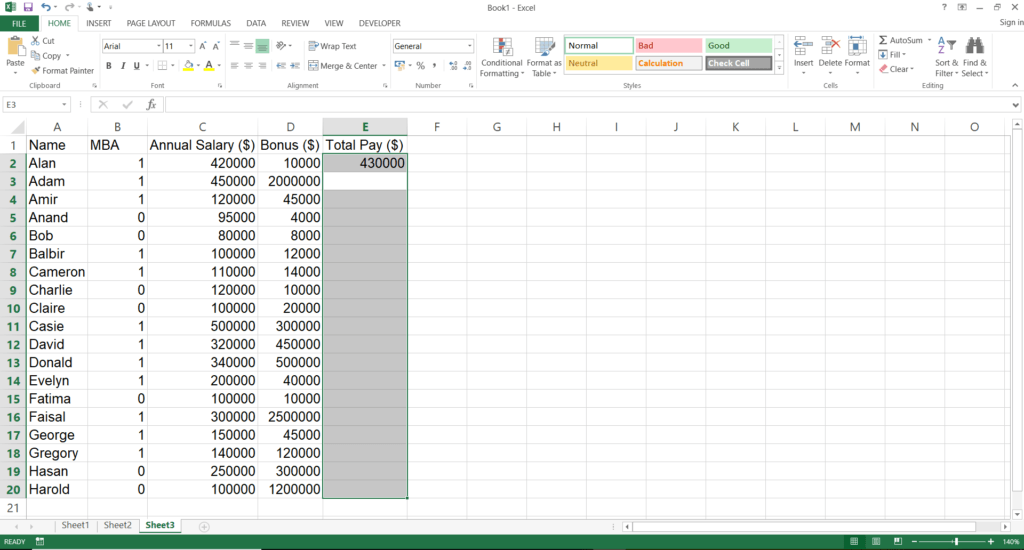5. We need to press the Control/Command, Shift, and Enter keys simultaneously.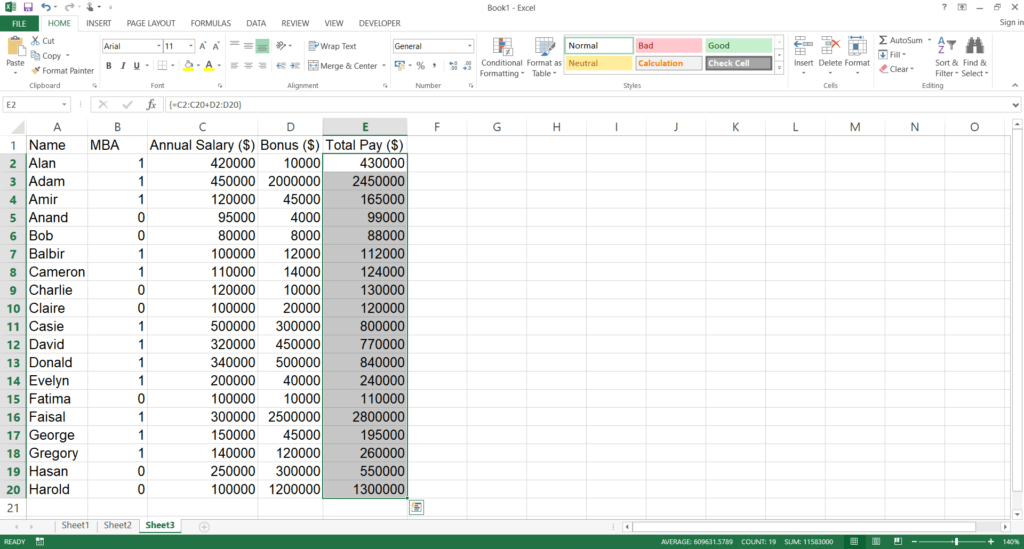### More Resources

CFI offers the Financial Modeling & Valuation Analyst (FMVA)™ certification program for those looking to take their careers to the next level. To keep learning and developing your knowledge base, please explore the additional relevant resources below:

• Excel Modeling Best Practices
• Formula vs Function
• Free Excel Crash Course
• List of Excel Functions

### Free Excel Tutorial

To master the art of Excel, check out CFI’s FREE Excel Crash Course, which teaches you how to become an Excel power user.  Learn the most important formulas, functions, and shortcuts to become confident in your financial analysis.

Launch CFI’s Free Excel Course now to take your career to the next level and move up the ladder!